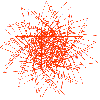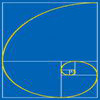Search by Topic

Resources tagged with Fibonacci sequence similar to Summats Clear:

Filter by: Content type:
Age range:
Challenge level:

There are 21 results

Broad Topics > Patterns, Sequences and Structure > Fibonacci sequencePythagorean Fibs

Age 16 to 18 Challenge Level:

What have Fibonacci numbers got to do with Pythagorean triples?Golden Fibs

Age 16 to 18 Challenge Level:

When is a Fibonacci sequence also a geometric sequence? When the ratio of successive terms is the golden ratio!Gnomon Dimensions

Age 14 to 16 Challenge Level:

These gnomons appear to have more than a passing connection with the Fibonacci sequence. This problem ask you to investigate some of these connections.Fibonacci Fashion

Age 16 to 18 Challenge Level:

What have Fibonacci numbers to do with solutions of the quadratic equation x^2 - x - 1 = 0 ?Farey Fibonacci

Age 16 to 18 Short Challenge Level:

Investigate Farey sequences of ratios of Fibonacci numbers.Plus or Minus

Age 16 to 18 Challenge Level:

Make and prove a conjecture about the value of the product of the Fibonacci numbers $F_{n+1}F_{n-1}$.Fibonacci Factors

Age 16 to 18 Challenge Level:

For which values of n is the Fibonacci number fn even? Which Fibonnaci numbers are divisible by 3?Building Gnomons

Age 14 to 16 Challenge Level:

Build gnomons that are related to the Fibonacci sequence and try to explain why this is possible.Golden Mathematics

Age 16 to 18

A voyage of discovery through a sequence of challenges exploring properties of the Golden Ratio and Fibonacci numbers.First Forward Into Logo 11: Sequences

Age 11 to 18 Challenge Level:

This part introduces the use of Logo for number work. Learn how to use Logo to generate sequences of numbers.Got a Strategy for Last Biscuit?

Age 11 to 16 Challenge Level:

Can you beat the computer in the challenging strategy game?Leonardo of Pisa and the Golden Rectangle

Age 7 to 16

Leonardo who?! Well, Leonardo is better known as Fibonacci and this article will tell you some of fascinating things about his famous sequence.Spirals Instead of Sunflowers

Age 11 to 16 Challenge Level:

Using logo to investigate spiralsLOGO Challenge - Circles as Bugs

Age 11 to 16 Challenge Level:

Here are some circle bugs to try to replicate with some elegant programming, plus some sequences generated elegantly in LOGO.Golden Powers

Age 16 to 18 Challenge Level:

You add 1 to the golden ratio to get its square. How do you find higher powers?Continued Fractions I

Age 14 to 18

An article introducing continued fractions with some simple puzzles for the reader.Golden Fractions

Age 16 to 18 Challenge Level:

Find the link between a sequence of continued fractions and the ratio of succesive Fibonacci numbers.The Golden Ratio, Fibonacci Numbers and Continued Fractions.

Age 14 to 16

An iterative method for finding the value of the Golden Ratio with explanations of how this involves the ratios of Fibonacci numbers and continued fractions.Ordered Sums

Age 14 to 16 Challenge Level:

Let a(n) be the number of ways of expressing the integer n as an ordered sum of 1's and 2's. Let b(n) be the number of ways of expressing n as an ordered sum of integers greater than 1. (i) Calculate. . . .Whirling Fibonacci Squares

Age 11 to 16

Draw whirling squares and see how Fibonacci sequences and golden rectangles are connected.Stringing it Out

Age 14 to 16 Challenge Level:

Explore the transformations and comment on what you find.